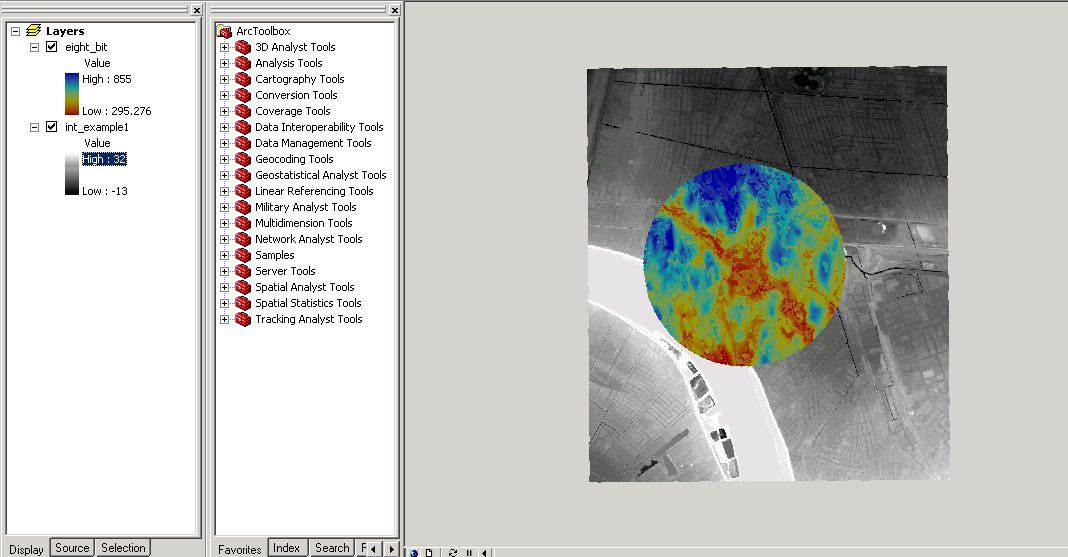English

How To: Add two rasters together and preserve all pixels

Summary

Instructions provided describe how to add two rasters together and preserve all pixel values from each raster dataset. Currently, when adding, subtracting, multiplying, dividing a raster by another raster, only the intersecting cells are preserved.

Procedure

Spatial Analyst is required for this procedure. The procedure assumes that the two rasters are not of the same extent.1. Go to ArcToolbox > Spatial Analyst Tools > Single Output Map Algebra.

2. Type the following expression using the smaller image as the input, changing the path of the raster accordingly:

CON(ISNULL(C:\Data\eight_bit), 0, (C:\Data\eight_bit))
3. Click 'Environments' at the bottom of this tool. Expand 'General Settings'.
4. Scroll down to 'Extent' and specify this as the larger raster.
5. Click OK > specify an output raster > click OK to run the tool. The output raster is the same size as the larger raster. The extended extent has pixel values of 0. Where pixels did not overlap before, now they do with a pixel value of 0.
6. Perform the mathematical operation using the output from the Single Output Map Algebra. Every pixel value is preserved from both raster datasets.